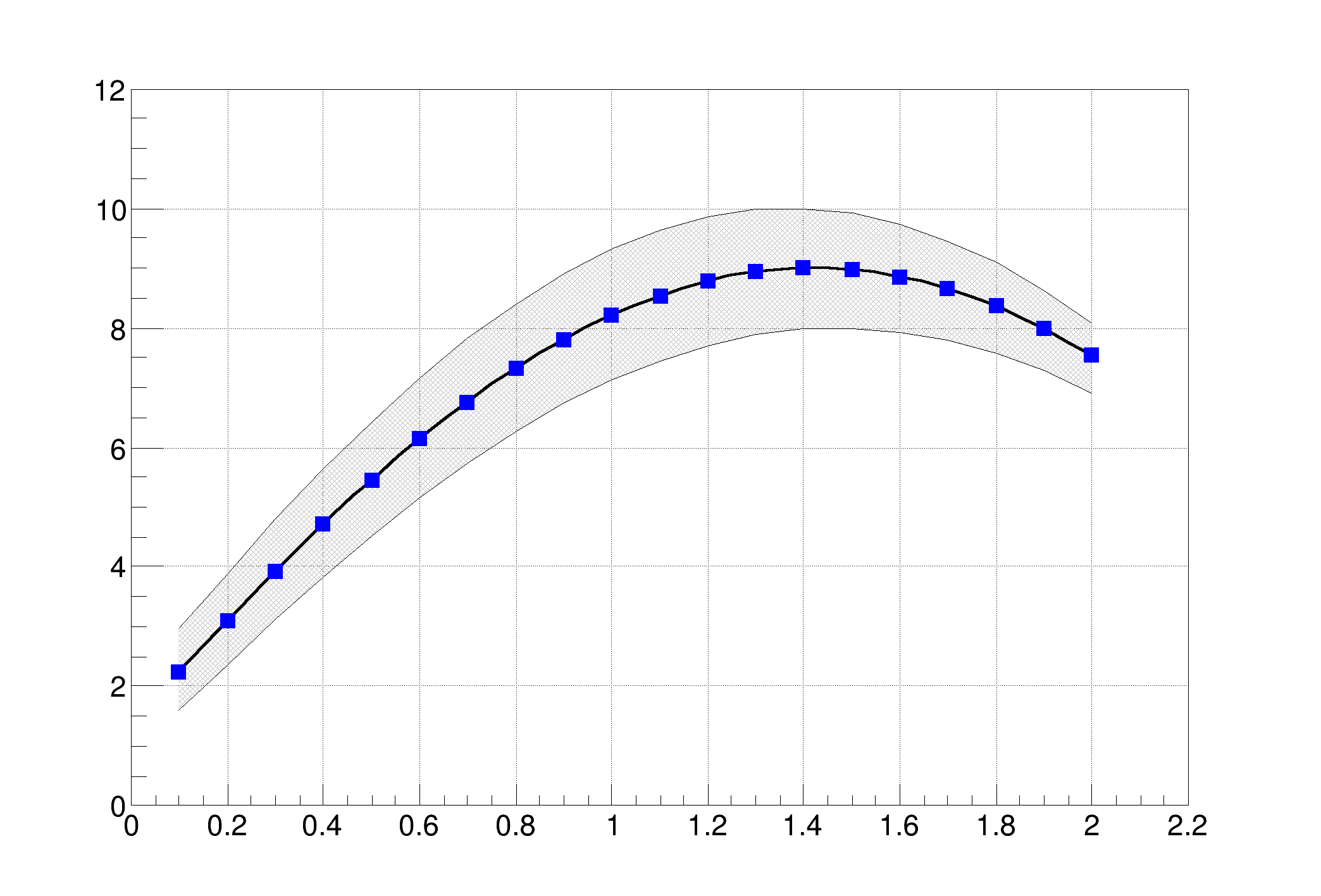ROOT   Reference Guide## Detailed DescriptionShow how to shade an area between two graphsTCanvas *c1 = new TCanvas("c1",
"A Simple Graph Example",200,10,700,500);
c1->SetGrid();
c1->DrawFrame(0,0,2.2,12);
const Int_t n = 20;
Double_t x[n], y[n],ymin[n], ymax[n];
Int_t i;
for (i=0;i<n;i++) {
x[i] = 0.1+i*0.1;
ymax[i] = 10*sin(x[i]+0.2);
ymin[i] = 8*sin(x[i]+0.1);
y[i] = 9*sin(x[i]+0.15);
}
TGraph *grmin = new TGraph(n,x,ymin);
TGraph *grmax = new TGraph(n,x,ymax);
TGraph *gr = new TGraph(n,x,y);
for (i=0;i<n;i++) {
}
grmin->Draw("l");
grmax->Draw("l");
gr->Draw("CP");
}
int Int_t
Definition: RtypesCore.h:45
double Double_t
Definition: RtypesCore.h:59
float ymin
Definition: THbookFile.cxx:95
float ymax
Definition: THbookFile.cxx:95
double sin(double)
virtual void SetFillColor(Color_t fcolor)
Set the fill area color.
Definition: TAttFill.h:37
virtual void SetFillStyle(Style_t fstyle)
Set the fill area style.
Definition: TAttFill.h:39
virtual void SetLineWidth(Width_t lwidth)
Set the line width.
Definition: TAttLine.h:43
virtual void SetMarkerColor(Color_t mcolor=1)
Set the marker color.
Definition: TAttMarker.h:38
virtual void SetMarkerStyle(Style_t mstyle=1)
Set the marker style.
Definition: TAttMarker.h:40
The Canvas class.
Definition: TCanvas.h:23
A TGraph is an object made of two arrays X and Y with npoints each.
Definition: TGraph.h:41
virtual void SetPoint(Int_t i, Double_t x, Double_t y)
Set x and y values for point number i.
Definition: TGraph.cxx:2284
virtual void Draw(Option_t *chopt="")
Draw this graph with its current attributes.
Definition: TGraph.cxx:769
return c1
Definition: legend1.C:41
Double_t y[n]
Definition: legend1.C:17
Double_t x[n]
Definition: legend1.C:17
const Int_t n
Definition: legend1.C:16
TGraphErrors * gr
Definition: legend1.C:25